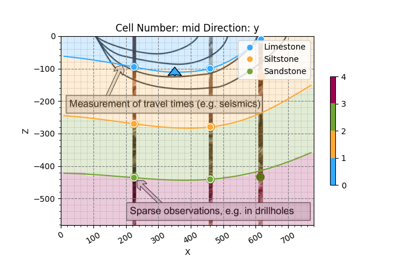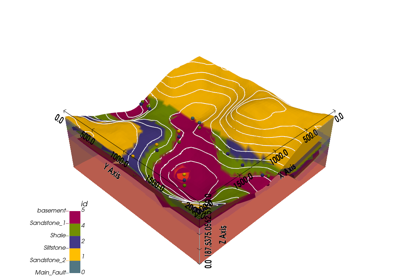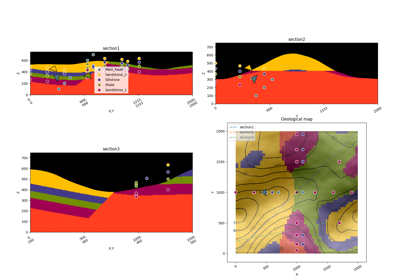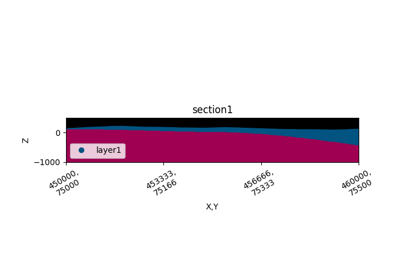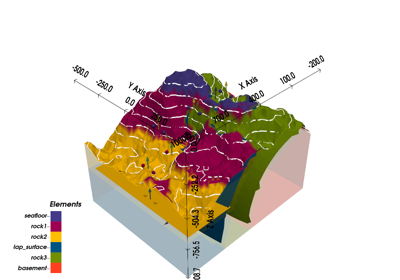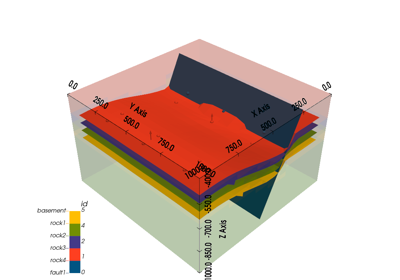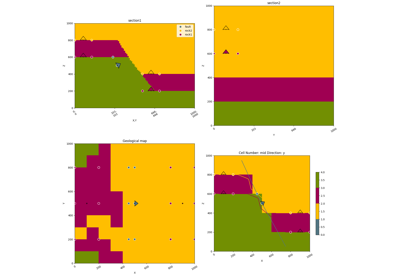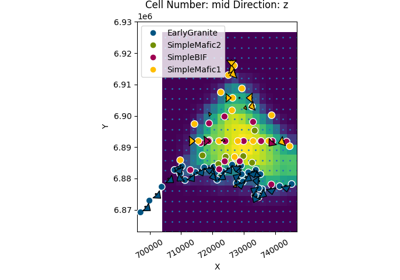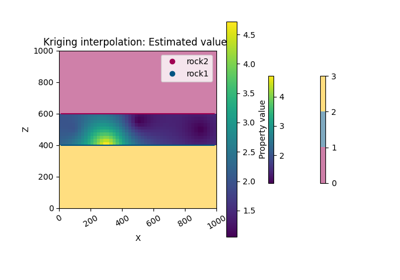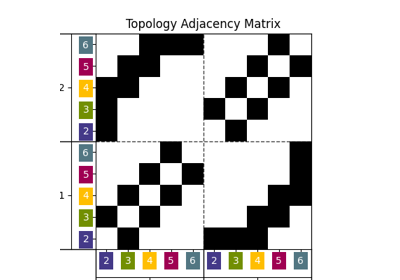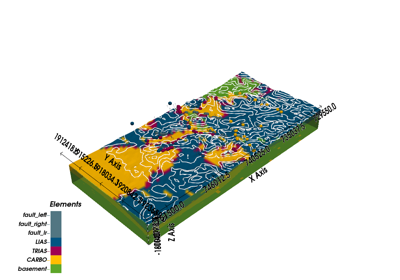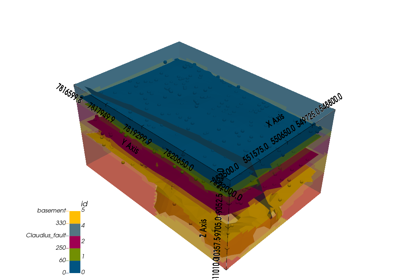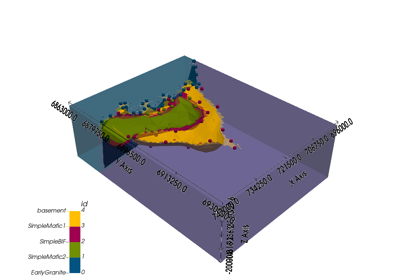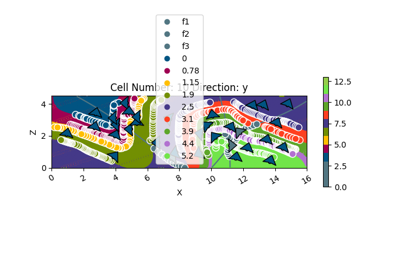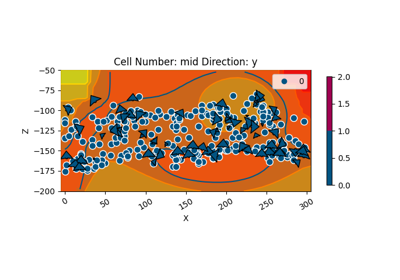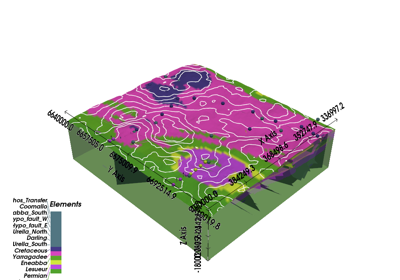# gempy.plot_2d¶

gempy.plot_2d(model, n_axis=None, section_names: Optional[list] = None, cell_number: Optional[list] = None, direction: list = 'y', show_data: Union[bool, list] = True, show_results: Union[bool, list] = True, show_lith: Union[bool, list] = True, show_values: Union[bool, list] = False, show_block: Union[bool, list] = False, show_scalar: Union[bool, list] = False, show_boundaries: Union[bool, list] = True, show_topography: Union[bool, list] = False, show_section_traces: Union[bool, list] = True, series_n: Union[int, List[int]] = 0, ve=1, block=None, regular_grid=None, kwargs_topography=None, kwargs_regular_grid=None, **kwargs)[source]

Plot 2-D sections of geomodel.

Plot cross sections either based on custom section traces or cell number in xyz direction. Options to plot lithology block, scalar field or rendered surface lines. Input data and topography can be included.

Parameters
• show_block (bool) – If True and model has been computed, plot cross section of the final model.

• show_values (bool) – If True and model has been computed, plot cross section of the value… TODO need to add attribute to choose which value to be plot

• model – Geomodel object with solutions.

• n_axis (int) – Subplot axis for multiple sections

• section_names (list) – Names of predefined custom section traces

• cell_number (list) – Position of the array to plot

• direction (str) – Cartesian direction to be plotted (xyz)

• show_data (bool) – Show original input data. Defaults to True.

• show_results (bool) – If False, override show lith, show_calar, show_values

• show_lith (bool) – Show lithological block volumes. Defaults to True.

• show_scalar (bool) – Show scalar field isolines. Defaults to False.

• show_boundaries (bool) – Show surface boundaries as lines. Defaults to True.

• show_topography (bool) – Show topography on plot. Defaults to False.

• series_n (int) – number of the scalar field.

• ve (float) – vertical exageration

• regular_grid (numpy.ndarray) – Numpy array of the size of model.grid.regular_grid

• kwargs_topography (dict) –

• fill_contour

• azdeg (float): azimuth of sun for hillshade

• altdeg (float): altitude in degrees of sun for hillshade

Keyword Arguments
• legend (bool) – If True plot legend. Default True

• show (bool) – Call matplotlib show

Returns

Plot2D object

Return type

gempy.plot.visualization_2d.Plot2D

## Examples using gempy.plot_2d¶